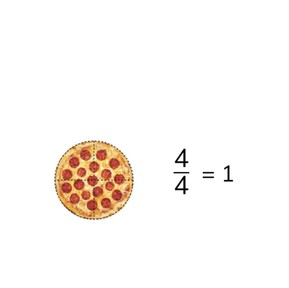Simplifying fractions greater than one to mixed numbers

# Simplifying fractions greater than one to mixed numbers

I can simplify fractions greater than 1 into mixed number.8,000 schools use Gynzy92,000 teachers use Gynzy1,600,000 students use Gynzy

## General

Students learn to simplify fractions greater than 1 into mixed numbers to make them easier to understand.

## Common core standard

CCSS.Math.Content.4.NF.B.3.B
CCSS.Math.Content.4.NF.B.3a

## Learning Objective

Students will be able to simplify fractions greater than 1 into mixed numbers.

## Introduction

Students are asked to form proper fractions using a fraction bar. They are then asked to determine which fraction is shown by a fraction bar.

## Instruction

Begin by reviewing the terms numerator and denominator to the students. Explain that the fractions can be simplified to show the largest possible number. The goal is to make the denominator as small as possible. Remind students that when the numerator and denominator are the same, you have a whole number. Explain to students that when the numerator is larger than the denominator, it is a fraction greater than 1 and that the number can also be simplified. When doing so, you end up with a mixed number, or a number which consists of whole number(s) and fractions. Show the steps to turn a fraction greater than 1 into a mixed number. Have students practice with an example and then determine if the fractions greater than 1 have been correctly converted into mixed numbers.

## Quiz

Students are asked a variety of questions, in which they must indicate if a fraction has been correctly simplified, to simplify a given fraction as much as possible, and to drag fractions greater than 1 to their mixed number equals.

## Closing

Discuss the importance of mixed numbers as a class, they are numbers that are better able to be understood in real world situations. Have the class drag the fractions greater than 1 to their correct mixed number.

## Teaching tips

Students who have difficulty can be helped by visualizing each fraction by drawing it out.

### The online teaching platform for interactive whiteboards and displays in schools

• Save time building lessons

• Manage the classroom more efficiently

• Increase student engagement